# An unsaturated hydrocarbon X on ozonolysis gives A.Question:

An unsaturated hydrocarbon $\mathrm{X}$ on ozonolysis gives $\mathrm{A}$. Compound $\mathrm{A}$ when warmed with ammonical silver nitrate forms a bright silver mirror along the sides of the test tube. The unsaturated hydrocarbon $X$ is:

1.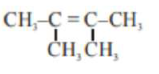2.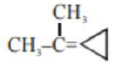3.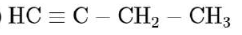4.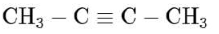Correct Option: , 3

Solution: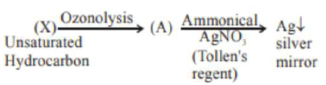As (A) compound given positive tollen's test hence it may consist - $\mathrm{CHO}$ (aldehyde group). or it can be $\mathrm{HCOOH}$ So for the given option:

(c)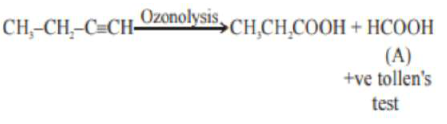and for other compounds (options):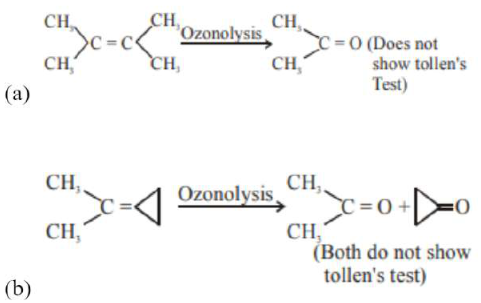(d) $\mathrm{CH}_{3}-\mathrm{C} \equiv \mathrm{C}-\mathrm{CH}_{3} \stackrel{\text { Ozonolysis }}{\longrightarrow} 2 \mathrm{CH}_{3}-\mathrm{COOH}$

(Does not show tollen's test)